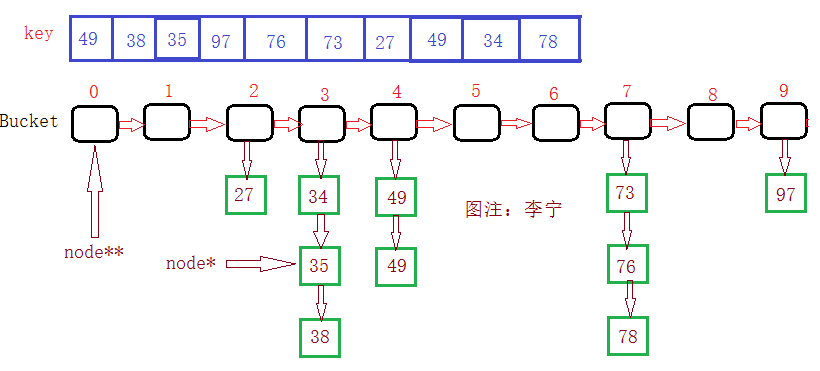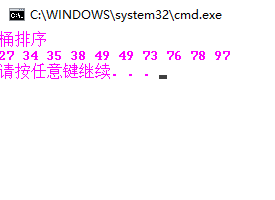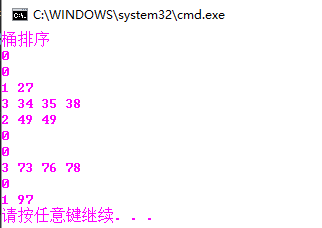# 浅显易懂的桶排序

1.假设：桶待排序列：{ 49, 38, 35, 97, 76, 73, 27, 49 ,34,78}2.关于映射及桶排原理分析：

桶中数据的十位都一样，那么映射函数算出的桶号就是这些数据的十位，也就是说，这种映射函数，目的就是为了找出数据的共同特点，可能是求余（哈希表直接定址，就是这样），可能是除某数求商，本例中就是取商；

#pragma once

//每一个节点的结构
struct node
{
int key;  //关键字，在桶中统计桶中数据量，在数据节点中就是节点的数据
struct node *next;
};

//声明：
void PrintBucketSort(node** bucket, int bucket_size);
int f(int x);

void BucketSort(int* a, int size,int bucket_size)
{
assert(a);

//给桶申请空间
node** bucket = new node*[bucket_size*sizeof(node)];

//初始化
for (int i = 0; i < bucket_size; ++i)
{
bucket[i] = new node[sizeof(node)]; //每一个桶
bucket[i]->key = 0;
bucket[i]->next = nullptr;
}

for (int j = 0; j < size; ++j)
{
node* sub_node = new node[sizeof(node)]; //桶下的每一个节点
sub_node->key = a[j];
sub_node->next = nullptr;

//计算这数据在哪个桶中
int num = f(a[j]);

//让一个指针指向这个桶号的头
node* sub_head = bucket[num];

//开始插入
if (sub_head->next == nullptr)
{
bucket[num]->next = sub_node;
bucket[num]->key++;
}
//该桶号不为空，那么插入排序
else
{
while (sub_head->next != nullptr && sub_node->key >= sub_head->next->key)
{
sub_head = sub_head->next;
}

sub_node->next = sub_head->next;
sub_head->next = sub_node;
bucket[num]->key++;
}
}
//打印
PrintBucketSort(bucket, bucket_size);
}

//映射函数
int f(int x)
{
return (x / 10);
}

//打印
void PrintBucketSort(node** bucket, int bucket_size)
{
//多少桶链(桶号)
for (int i = 0; i < bucket_size; ++i)
{
node* cur = bucket[i]->next;
while (cur)
{
cout << cur->key << " ";
cur = cur->next;
}
}
cout << endl;
}

void Test7()
{
int a = { 49, 38, 35, 97, 76, 73, 27, 49, 34, 78 };
cout << "桶排序" << endl;
BucketSort(a, 10, 10);  //桶数据最大才97，所以需要10个桶
}O(N)+O(M*(N/M)*log(N/M))=O(N+N*(logN-logM))=O(N+N*logN-N*logM)

09-22129604-014万+
06-231万+
09-11979
12-03482
04-221640
03-223090
08-1336
01-20682
03-163505
05-02893
04-19577
04-08499
05-281万+
09-171万+
©️2020 CSDN 皮肤主题: 编程工作室 设计师:CSDN官方博客